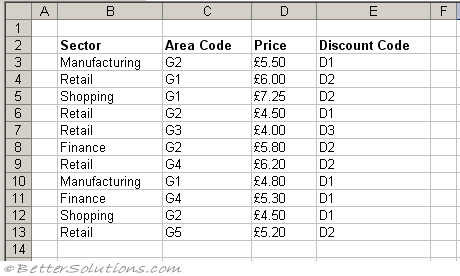# Lookup Multiple Criteria

A quick solution is to add an additional column which is a concatenation of the columns you want to match
The formulas below can be used when you do not want to insert an additional column.
returns a value in the same row after finding matching values in two columns

### Additional Column (large data sets)

For very large data sets you should always insert an additional (helper) column to avoid performance problems.
vlookup(concatenate())

### Two Criteria - IF Function

Lets assume we have got the following table and we want to return the Price corresponding to the sector "Retail" and the area code "G3".={INDEX(D3:D13,MATCH("Retail",IF(C3:C13="G3",B3:B13),0))} =

### Two Criteria - String Concatenation

This is often a preferred method as it does not include nested IF functions.

 ={INDEX(D3:D13,MATCH("Retails"&"G3",B3:B13&C3:C13,0))} =

### Two Column Lookup

The MATCH function can be used to lookup values in a table based on matching values in two columns.
The MATCH function returns the position of a value in a list

### Two Column Matching

Lets consider the following table where we want to return the department of a specific individual, matching on first name and last name
{MATCH("David" & "Smith",B3:B14&C3:C14,0)} = 4
Once we know which row matches we can combine this function with the INDEX function to return the intersection of this row with the column containing the department

{=INDEX(D3:D14,MATCH("David" & "Smith",B3:B14&C3:C14,0) ) }

### INDEX and MATCH

=INDEX( MATCH( ) )
=INDEX( MATCH( ), MATCH( ) )
To make this type of two way lookup simpler to maintain you might want to create a named range for the whole table and then use a formula that refers to that named range.
Lets assume you have the following table and the highlighted cells have the named range "NamedRange".
SS - above with cells highlighted
=INDEX( MATCH( INDEX() ), MATCH( INDEX() ) )
={INDEX(NamedRange, MATCH("Leeds", INDEX(NamedRange,,1),0),MATCH("Bristol",INDEX(NamedRange,1),))}

### Two Critera - Multiplication

 ={INDEX(D3:D13,MATCH(1,("B3:B13="Retail")*(C3:C13="G3"),0))}

### Three Criteria - IF Function

are these embedded ?
do you have to use an embedded if ?